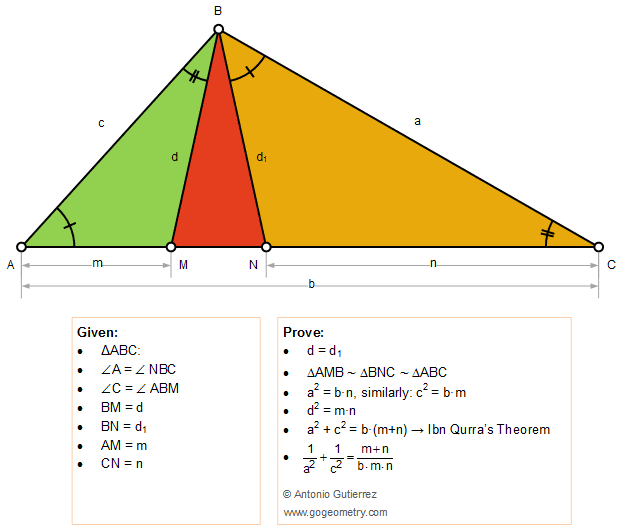# Geometry Problem 1386: Thabit ibn Qurra (826 -901) Theorem and more conclusions, generalized Pythagorean Theorem to any triangle

### Proposition

The figure below shows a triangle ABC of sides a, b, c with the cevians BM and BN so that the angles A and C are congruent to the angles NBC and ABM, respectively. BM = d, BN = d1, AM = m, and CN = n. Prove that  (1) triangle MBN is isosceles: d = d1;  (2) triangles AMB , BNC and ABC are similar;  (3) a2 = b.n, similarly c2 = b.m;  (4) d2 = m.n;  (5) a2 + c2 = b.(m+n) Qurra's theorem;  (6) 1/a2 + 1/c2 = (m+n)/(b.m.n).### Geometric Art: Hyperbolic Kaleidoscope of problem 1386